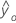# Methods and formulas for Predict for Stability Study for fixed batches

Select the method or formula of your choice.

## GLM model

In matrix terms, this is the formula for the general linear regression model:

### Notation

TermDescription
Yvector of responses
Xdesign matrix
βvector of parameters
εvector of independent normal random variables

## Fitted value and SE fit for fixed batch

The fitted value is the predicted y or, which is the mean response value for the given predictor values using the estimated regression equation.

The standard error of the fitted value in a regression model with one predictor is:
The standard error of the fitted value in a regression model with more than one predictor is: where

### Notation

TermDescription
xiith predictor valuemean predictor
Xdesign matrix
nnumber of observations
s2mean square error

## Prediction interval

The range in which the predicted response for a new observation is expected to fall. The formula is:

### Notation

TermDescriptionfitted response value for a given set of predictor value
αlevel of significance
nnumber of observations
pnumber of terms in the model, including the intercept term if it is in the model
s2mean square error
xpredictor matrix
xomatrix of given predictor values beginning with a column of 1s when the intercept term is in the model

## Confidence interval

The range in which the estimated mean response for a given set of predictor values is expected to fall. The formula is:

### Notation

TermDescriptionfitted response value for a given set of predictor values
αlevel of significance
nnumber of observations
pnumber of terms in the model, including the intercept term if it is in the model
s2(b)variance-covariance matrix of coefficient
s2mean square error
Xpredictor matrix
Xomatrix of given predictor values beginning with a column of 1s when the intercept term is in the model
By using this site you agree to the use of cookies for analytics and personalized content.  Read our policy